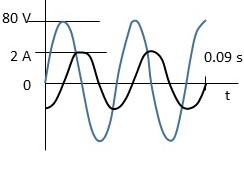# The voltage across and the current through a single circuit element connected to an ac generator...

## Question:

The voltage across and the current through a single circuit element connected to an ac generator are shown in the graph.a. At what frequency do charges oscillate in this circuit?

b. Determine the rms current through this circuit element.

## Frequency & Time Period:

The frequency can be defined as the number of repetitions/cycles completed by a wave in a unit time.

It is indirectly proportional to the time period and is given as -

{eq}\displaystyle F = \frac{1}{T}................(\text{where T is the time period in seconds})\\ {/eq}

Become a Study.com member to unlock this answer! Create your account

(a) Frequency -

{eq}\displaystyle F = \frac{1}{T}\\ \displaystyle F = \frac{1}{0.04}................(\text{As time is 0.04 sec for one completet...